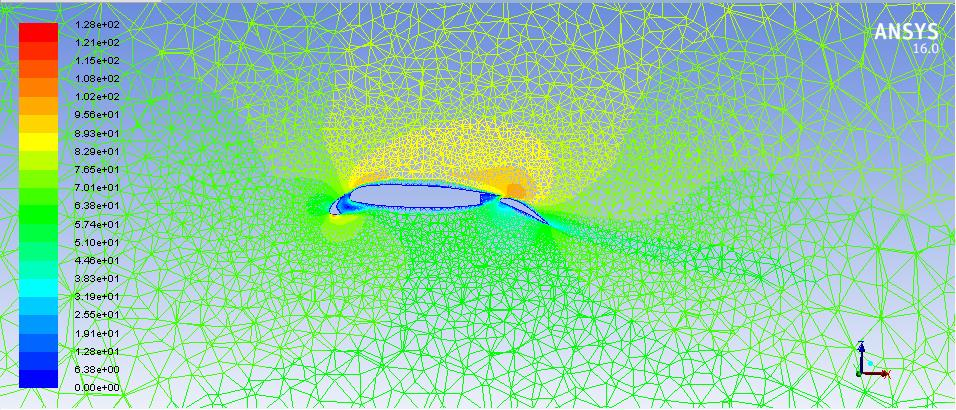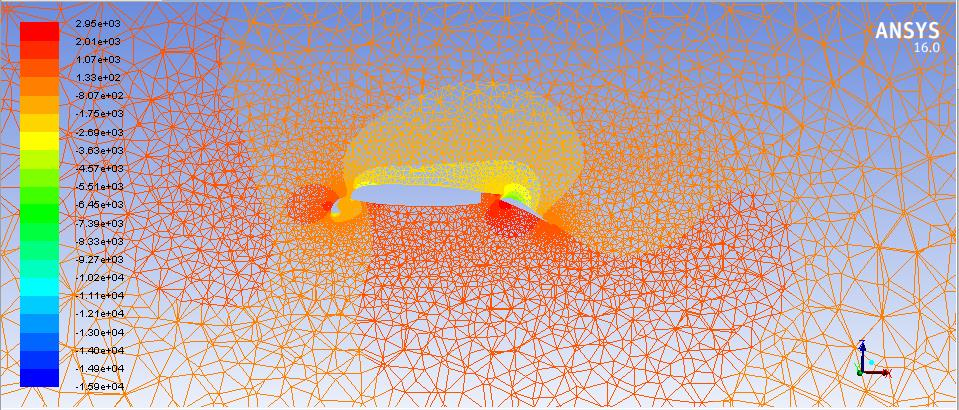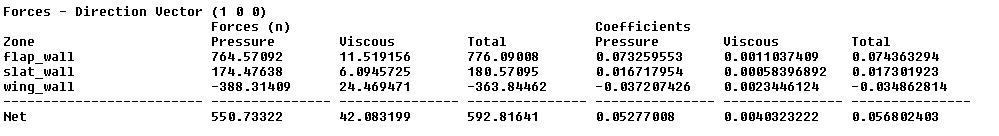# How to check Lift and drag values for a body which has Wing Slats and Flaps?

Hello,So I need to check Lift, Drag, Lift-coefficient and Drag coefficient for the model which looks like the above-attached image.

I did name all the three sections as Slat_wall, wing_wall, and Flap_wall respectively and in reference values, I added the area of all the three walls.

Now to check the Lift across the Whole model In results I selected all the three walls (slat, wing, flap) and then checked the force in the Z direction for lift and X direction for Drag, Is it the correct method to calculate lift and drag across the whole model?

Also, Does co-efficient of pressure mean Co-efficient of lift when I'm checking in Z direction?

P.S: All the 3 walls are not attached.

• edited July 2019
It depend how the values you compare with or you refer to are gathered or measured. Your appriach sounds rational. Check reference values for coefficients calculation and check if the direction vectors for lift and drag are really perpendicular or parallel respectively to inflow direction.
• It depend how the values you compare with or you refer to are gathered or measured. Your appriach sounds rational. Check reference values for coefficients calculation and check if the direction vectors for lift and drag are really perpendicular or parallel respectively to inflow direction.

Yes, the lift and drag vectors are perpendicular and parallel, respectively.

By asking me to check reference values are you asking me to check areas and calulate co-efficients by lift and drag equations?

• edited July 2019

Yes. Best to get the reference values from inflow zone

•When I check for forces, I get these values, can you tell me what doe coefficients of Pressure and total mean?

• edited July 2019

Pressure coefficients and drag coefficients. All these information is documented. Kind of dimensionsless groups made by calculating the ratio of the forces to dynamic pressure at inflow.